# 0708-1300/Hawaiian Earring

(diff) ← Older revision | Latest revision (diff) | Newer revision → (diff)
Assume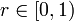$r\in[0,1)$ and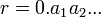$r=0.a_1a_2...$ is a decimal expansion without an infinite tail of nines. Let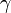$\gamma$ be a path that goes around the first (the largest) circle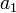$a_1$ times, then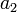$a_2$ times around the second and so on. Observe that thanks to the shrinking of the circles it is possible the continuity of the curve at time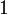$1$ for the irrational numbers. Proving that two such curves belongs to different homotopy classes tell us that the number of homotopy classes is uncountable.
Now, assume that two such curves, corresponding to two different real numbers, are homotopic. Let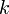$k$ be the first decimal digit in which the numbers differ and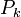$P_k$ the quotient map of identifying all the circles but the$k$-th one to the joint point. if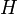$H$ is a homotopy of the curves then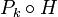$P_k\circ H$ is a homotopy of the projection of the curves which winds a different number of times. But this contradict the computation of the fundamental group o the circle.